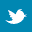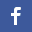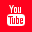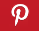Completely Solved C, C++ Programs Assignment.Quick Search Database Videos Tutorials Ebooks Forums FAQ Aboutus Household Industrial Manufacturing Service Shopping Transportation### C program to implement Prim's algorithm which generates a minimal spanning tree of a weighted connected graph given as input.

 Filed Under: C Assignments

Program Statement :
Write a C program to implement Prim's algorithm which generates a minimal spanning tree of a weighted connected graph given as input.

Theory :
In computer science, Prim's algorithm is an algorithm that finds a minimal spanning tree for a connected weighted graph. This means it finds a subset of the edges that forms a tree that includes every vertex, where the total weight of all the edges in this newly constructed tree is minimized. Prim's algorithm is an example of a greedy algorithm. It constructs minimal spanning tree considering vertices of the graph one by one and any one vertex can be chosen as the starting vertex.
To implement this algorithm ,a graph is taken as input in the form of a vertex by vertex matrix such that:
● w(i,j) = 0 if vertices vi and vj has no path between them and
if vi and vj are connected by an edge then
● w(i,j) = weight of that edge.
We also need a selected[] array which keeps track of the state of the vertices.
● selected[i] = False denotes ith vertex is not yet visited or selected.
● selected[i] = True denotes ith vertex is already visited.
The limitation of this algorithm is it cannot generate all possible minimal spanning trees for a given graph.

Algorithm :
/* The input graph,number of vertices and the starting vertex are passed as parameters */
Algo_prim(a[size][size],n,vs)
{
Initially no vertices are included in selected[];
for(i = 1 to n)
{
for(j = 1 to n)
tree[i][j] = 0; /*Initially spanning tree is empty*/
}
vs is included in selected[]; /*Starting vertex is selected at first*/
while(all the vertices are not selected)
{
min=infinity;
for(i=1 to n)
{
{
for(j = 1 to n)
{
if(jth vertex is not already selected)
{
if(there is a path between ith and jth vertex)
{
/*Search for an edge with minimum weight*/
if(min>a[i][j])
{
min=a[i][j];
x=i;
y=j;
}
}
}
}

}
}
/*Updation of previous cost by adding it to cost of newly selected edge*/
cost = cost+min;
/*The newly selected edge is included in the minimal spanning tree*/
tree[x][y] = min;
selected[y] = True;
}
print(tree);
return cost;
}/* End of Algo_prim */

Program listing :
/*C program to implement Prim's algorithm which generates a minimal spanning tree of a weighted connected graph given as input*/

#include<stdio.h>
#define inf 9999
#define size 10/*Defining maximum number of vertices of the input graph*/
#define True 1
#define False 0
void main()
{
int a[size][size],i,j,n,vs,min_weight;
int prim(int[][j],int,int);
printf("Enter the number of vertex : ");
scanf("%d",&n);
printf("Enter a weighted matrix(with weights) as input : n");
for(i=0;i<n;i++)
{
for(j=0;j<n;j++)
{
printf("Enter the value of a[%d][%d] : ",i,j);
scanf("%d",&a[i][j]);
}
}
printf("The entered matrix is : n");
for(i=0;i<n;i++)
{
for(j=0;j<n;j++)
printf("%dt",a[i][j]);
printf("n");
}
printf("Enter starting vertex: v");
scanf("%d",&vs);/*Read the starting vertex from user*/
if(vs<0||vs>n-1)/*Checking validity of the starting vertex given by the user*/
{
printf("!!!!!ERROR!!!!!n");
printf("!!!!!Invalid vertex given!!!!!");
return;
}
printf("nSelected order of edges : ");
min_weight=prim(a,n,vs); //call the prim function
printf("n");
printf("Minimum weight :%d",min_weight);/*The total weight of the minimal spanning tree is displayed in the output*/
}
/*The input graph,number of vertices and the starting vertex are passed as parameters*/
int prim(int a[size][size],int n,int vs)
{
int selected[size],tree[size][size],nv,i,j,x,y,cost=0,min;
for(i=0;i<n;i++)
selected[i]=False;/*Initially no vertices are selected*/
for(i=0;i<n;i++)
{
for(j=0;j<n;j++)
tree[i][j]=0;/*Initially spanning tree is empty*/
}
selected[vs]=True;/*Starting vertex is selected at first*/
nv=1;
while(nv<n)/*Iteration will be considered until all the vertices are selected*/
{
min=inf;/*min is initialized by a large value*/
for(i=0;i<n;i++)
{
if(selected[i]==True)/*Iteration will be considered iff i th vertex is already selected*/
{
for(j=0;j<n;j++)
{
if(selected[j]==False)/*Iteration will be considered iff j th vertex is not already selected*/

{
if(a[i][j]!=0)/*Iteration will be considered iff there is a path between i th and j th vertex*/
{
if(min>a[i][j])/*Search for an edge with minimum weight*/
{
min=a[i][j];
x=i;
y=j;
}
}
}
}
}
}
cost=cost+min;/*Updation of previous cost by adding it to cost of newly selected edge*/
tree[x][y]=min;/*The newly selected edge is included in the minimal spanning tree*/
selected[y]=True;
nv++;
printf("(v%d,v%d)->",x,y);
}
printf("b bb ");
printf("n");
printf("nThe spanning tree is(with weights) : n");
for(i=0;i<n;i++)
{
for(j=0;j<n;j++)
printf("%dt",tree[i][j]);
printf("n");
}
return cost;
}

Output (FOR GRAPH G):
Enter the number of vertex : 6
Enter a weighted matrix(with weights) as input :
Enter the value of a : 0
Enter the value of a : 3
Enter the value of a : 11
Enter the value of a : 0
Enter the value of a : 0
Enter the value of a : 0
Enter the value of a : 3
Enter the value of a : 0
Enter the value of a : 5
Enter the value of a : 4
Enter the value of a : 2
Enter the value of a : 0
Enter the value of a : 11
Enter the value of a : 5
Enter the value of a : 0
Enter the value of a : 1
Enter the value of a : 0
Enter the value of a : 0
Enter the value of a : 0
Enter the value of a : 4
Enter the value of a : 1
Enter the value of a : 0
Enter the value of a : 10
Enter the value of a : 8
Enter the value of a : 0
Enter the value of a : 2
Enter the value of a : 0
Enter the value of a : 10
Enter the value of a : 0
Enter the value of a : 9
Enter the value of a : 0
Enter the value of a : 0
Enter the value of a : 0
Enter the value of a : 8
Enter the value of a : 9
Enter the value of a : 0
The entered matrix is :
0 3 11 0 0 0
3 0 5 4 2 0
11 5 0 1 0 0
0 4 1 0 10 8
0 2 0 10 0 9
0 0 0 8 9 0
Enter starting vertex: v4
Selected order of edges : (v4,v1)->(v1,v0)->(v1,v3)->(v3,v2)->(v3,v5)
The spanning tree is(with weights) :
0 0 0 0 0 0
3 0 0 4 0 0
0 0 0 0 0 0
0 0 1 0 0 8
0 2 0 0 0 0
0 0 0 0 0 0
Minimum weight :18

Discussions :
● The time complexity of Prim's algorithm (using simple weighted matrix form) is O(n2), where n is the number of vertices of the graph.
● Using a simple binary heap data structure and an adjacency list representation, Prim's algorithm can be shown to run in time O(| E | log | V |) where | E | is the number of edges and | V | is the number of vertices. Using a more sophisticated Fibonacci heap, this can be brought down to O(| E | + | V | log | V |), which is significantly faster when the graph is dense enough that | E | is Ω(| V |).
● Prim's algorithm can also be used for generating maximal spanning tree of a weighted connected graph. In that case edges with maximum weights are to be included in the spanning tree.
● Minimal or maximal spanning tree can also be generated by Kruskal's algorithm which generates such a tree by including edges one by one, whereas Prim's algorithm considers vertices one by one.
● Prim's algorithm has less overhead than Kruskal's algorithm.
● Prim’s algorithm is advantageous over Kruskal’s algorithm in finding the shortest spanning tree in a graph. It is faster and more convenient to use Prim’s algorithm than Kruskal’s.Back to main directory:Software Practical